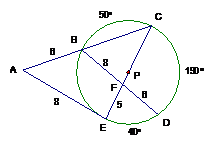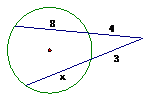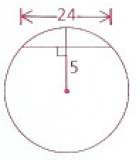Chapter 10 Circles Review
starstarstarstarstarstarstarstarstarstar
by Jennifer Webb
| 30 Questions
Note from the author:
Chapter 10 Circles Review1
2 pts
In the circumscribed quadrilateral, find CD
2
2 pts
Circle O has a radius of 25 meters and a chord of 48 meters. How far is the chord from the center of the circle?
3
2 pts
Circle P has a radius of 7 in and circle O has a radius of 1 in. The distance from their centers is 10 cm. Find the length of the common external tangent.4
2 pts
Given: BA and BC are tangent to circle D. The ratio of arc AFC to arc AC 4:1. Find measure of angle B.
5
2 pts
A circle with center (-4,1) passes through (3,-6). Find the slope of the tangent line through (3,-6)6
2 pts
Find measure of angle A7
2 pts
Find length of segment FC
8
2 pts
A square with an area of 81 is inscribed in a circle. Find the circumference of the circle.9
2 pts
Find the measure of angle D10
2 pts
Find the measure of angle A
11
2 pts
Circle X with radius 9 cm and Circle Y with radius 6 cm are 19 cm apart. Find the length of the common external tangent.
12
2 pts
Circle P with a radius 12 cm and Circle Q with a radius of 7 cm. Find the distance between the centers if the common external tangent segment is 15 cm.
13
2 pts
Two concentric circles have radii 6 and 10. Find the length of a chord of the larger circle that is tangent to the smaller circle.14
2 pts
In the circumscribed quadrilateral, find CD15
2 pts
Solve for x16
2 pts
Solve for x17
2 pts
Solve for x18
2 pts
Solve for x19
2 pts
Solve for x20
2 pts
Solve for x21
2 pts
Solve for x22
2 pts
Find segment BD23
2 pts
Find length of PT24
2 pts
Find length of WX25
2 pts
Find the radius of the circle26
2 pts
Find the radius of cirle C
27
2 pts
A quadrilateral is inscribed in a circle. Its vertices divide the circle into four arcs in the ratio 1:2:5:4. Find the largest angle.28
2 pts
In the given circle, arc AB is 30, arc BC is 40, and arc CD is 50. Find the measure of angle X
29
2 pts
In the given circle, arc AB is 30, arc BC is 40, and arc CD is 50. Find the measure of angle Y
30
2 pts
In the given circle, arc AB is 30, arc BC is 40, and arc CD is 50. Find the measure of angle Z